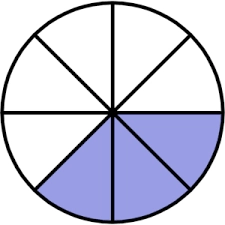# My fraction

My fraction is not a mixed number. My fraction is equivalent to 1/2. When my fraction is divided by 1/3, the answer is 1 1/2. The numerator of the fraction is 4. What is my fraction?

x =  1/2

### Step-by-step explanation:Did you find an error or inaccuracy? Feel free to write us. Thank you!

Tips for related online calculators
Need help calculating sum, simplifying, or multiplying fractions? Try our fraction calculator.